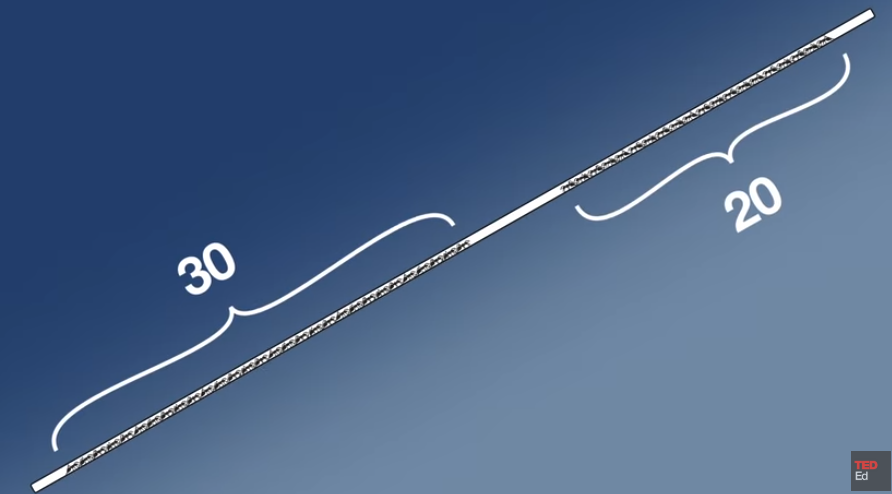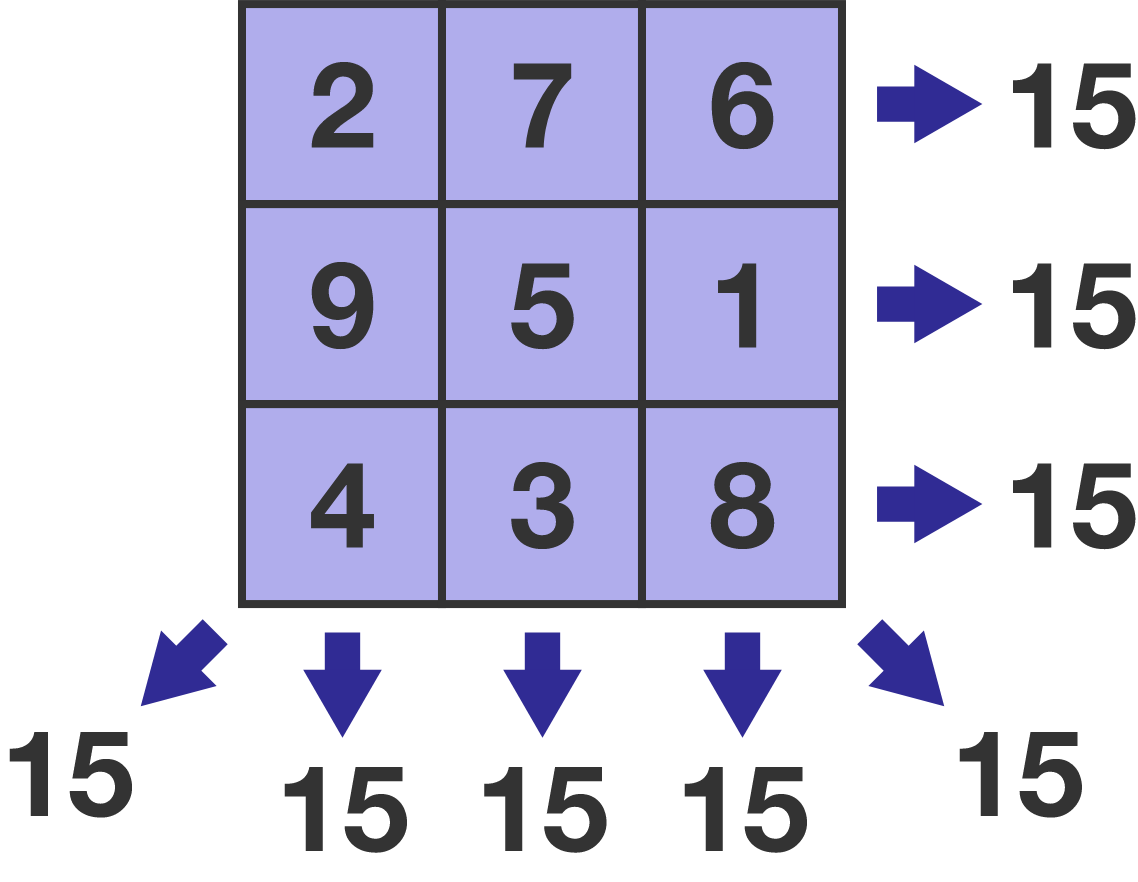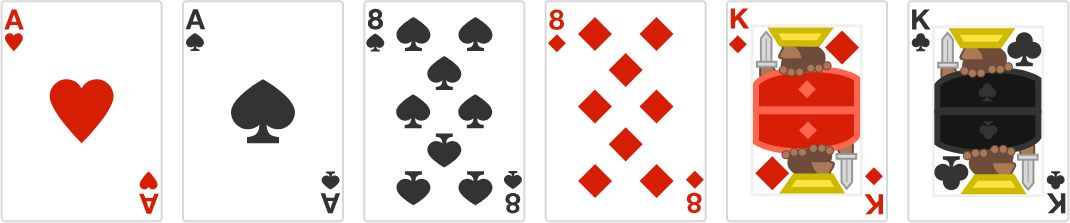# Problems of the Week

Contribute a problem

# 2018-10-08 Intermediate

Suppose you have a single tube with 30 roboants on one end and 20 roboants on the other.All the roboants on each side start out marching in a line at a uniform pace towards the other end of the tube. When two roboants bump into each other, they instantly turn around and walk back the way they came.

How many total ant collisions will there be?The animation is courtesy TED-Ed and Artrake Studio.

Note: When the roboants reach either end of the tube, they exit the tube.A magic square consists of a $3\times 3$ grid filled in with the digits 1-9 such that every column, row, or diagonal adds up to the same constant (typically 15).

Is it possible to fill each box with a distinct integer such that the ${\color{#D61F06} \textbf{product}}$ of every column, row, or diagonal is the same number?

Note: The integers don't need to be the numbers 1-9.

All the real solutions of $\sqrt{\sqrt{x+5}+5} = x$ can be written in the form $\frac{a+\sqrt{b}}{c},$ where $a,$ $b,$ and $c$ are positive integers and $b$ is square-free.

What is the total sum of $a+b+c$ for all of the solutions?

Suppose you arrange some cards from a standard 52-card poker deck with the following conditions:

• Arrange the cards side by side in a line.
• Any two adjacent cards are either the same suit or rank.
• No three cards in a row are the same suit or rank.

What is the maximum number of cards that can be arranged like this?The image illustrates the first 6 cards of such an arrangement.

You do a load of laundry containing $4$ identical black socks, $4$ identical blue socks, and $4$ identical white socks. When it is finished, you randomly place all the socks in a single row on a table so that you can match them later on.

To your surprise, all $6$ pairs of socks are already beside their match!

The probability that a match-up is already complete is $\frac{1}{x}.$

What is $x?$×